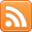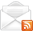# ASP.NET,C#.NET,VB.NET,JQuery,JavaScript,Gridview

aspdotnet-suresh offers C#.net articles and tutorials,csharp dot net,asp.net articles and tutorials,VB.NET Articles,Gridview articles,code examples of asp.net 2.0 /3.5,AJAX,SQL Server Articles,examples of .net technologies

### How to get length of data in each Column of a table Using SQL Server

Sep 4, 2010
Introduction:

Here I will explain how to get length of data in each Column of a table using SQL Server.

Description:

There is sometimes we need to figure out the maximum space that is being used by a particular column in your database.  You would initially think that the LEN() function would allow you to do this, but this function does not work on Text, NText or Image data types, so how do you figure out the length of a value in a column that has one of these data types?

In addition to the LEN () function, SQL Server also has a DATALENGTH () function.  This function can be used on all data types in your table.

Here is an example for DATALENGTH () function:

 /* Sample to find the length of Data in each Column of a Table*/ SELECT DATALENGTH(NAME) as 'Name',DATALENGTH(VALUE) AS 'Record Value', DATALENGTH(TYPE) AS 'Type' FROM Sample_table
If you wanted to find out the maximum length used in Columns you could issue a command such as the following:

 /* Sample to find the Maxmimum length data in each Column of Table*/ SELECT MAX(DATALENGTH(NAME)) as 'Name',MAX(DATALENGTH(VALUE)) AS 'Record Value', MAX(DATALENGTH(TYPE)) AS 'Type' FROM Sample_table

 If you enjoyed this post, please support the blog below. It's FREE! Get the latest Asp.net, C#.net, VB.NET, jQuery, Plugins & Code Snippets for FREE by subscribing to our Facebook, Twitter, RSS feed, or by email.Subscribe by RSSSubscribe by Email